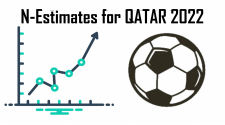# Sports Rating Algorithm Predicts FIFA World Cup 2022 Knockout StagesQuintessa mathematicians and scientists enjoy analysing numbers. Recently, Simon Rookyard and Jodie Stone presented predictions of the results in the group stage of the ongoing FIFA World Cup - Qatar 2022. With the group stage complete, they now present predictions for the round of 16.

In a recent article, we used our "N-Estimates" algorithm to predict the 48 opening round matches of FIFA World Cup - Qatar 2022. We have updated the teams’ ratings following the first round results and our predictions for the remaining rounds of the competition are below.

The prediction plots (given below) indicate the likelihood levels of various possible results. The x- and y- axes represent potential numbers of goals scored by each team. The team on the x-axis wins if the predicted scoreline is below the blue dashed line and the y-axis team wins when above (a predicted scoreline on the blue dashed line is hence a draw).

The colour of the plotted points denotes the probability of each given scoreline – this is scaled, with a high probability plotted in orange and a low probability in blue. The plotted scoreline embellished with a cross denotes the most likely result. The solid orange line represents the most likely difference in performance between the teams during the match, expressed as a goal difference (as this is based on non-integer mean ratings for the two teams, it is not necessarily an integer value). The 1σ uncertainty range for this predicted goal difference is given by the two dashed orange lines.

See Figure 1 for predictions for the first six matches of the round of 16. Future matches will be added when the competing teams are known.Figure 1: Predictions for the round of 16. For each plot, the circles represent possible final scores after 90 minutes, with the number of goals scored by each team plotted on the axes. Each circle has been colour coded to indicate the probability of that result occurring, with the most likely outcome marked with a black cross. The solid and dashed orange lines represent the mean and 1σ uncertainty range respectively for the predicted goal difference, and the dashed blue lines indicate a goal difference of zero.